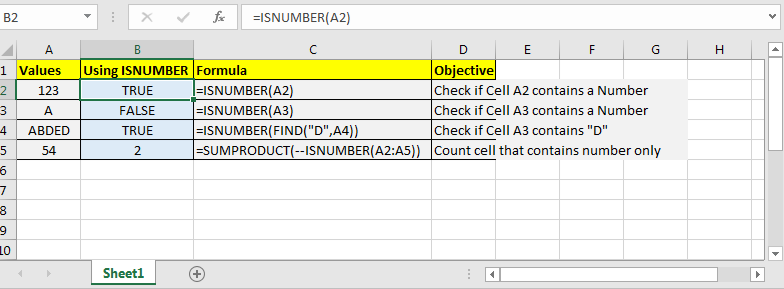# How to Use ISNUMBER Function in Excel

Excel’s ISNUMBER function is a boolean function that returns TRUE or FALSE if supplied value is a number or not respectively.Syntax

=ISNUMBER(value)

It simply take one argument and returns TRUE if its a number else FALSE. Most of the time you will passing references of a cell or a formula as values to check that if its a number or not.

#### Examples:

• Check if Cell A2 contains a number or not
=ISNUMBER(A2)
• Check If formula returns a number or not
=ISNUMBER(FIND(“A”,A2))
• Count cells that contains number.You can pass whole range to ISNUMBER function. It will show if only first element is number or not but internally it will store an array of true and false. It come in handy while working with excel formulas that accept arrays as input.
=SUMPRODUCT(--(ISNUMBER(A2:A10)))

In many tutorial I have used ISNUMBER function to count or find out if a value is a number or not. You can check our articles Check If Cell Contains Specific Text and Count Cells that Contain Specific Text. I have used ISNUMBER function in these articles.

So yeah, this is what ISNUMBER do. Its simple yet powerful if used with other formula smartly.

Popular Articles:

50 Excel Shortcuts to Increase Your Productivity

How to use the VLOOKUP Function in Excel

How to use the COUNTIF function in Excel 2016

How to Use SUMIF Function in Excel

Terms and Conditions of use

The applications/code on this site are distributed as is and without warranties or liability. In no event shall the owner of the copyrights, or the authors of the applications/code be liable for any loss of profit, any problems or any damage resulting from the use or evaluation of the applications/code.